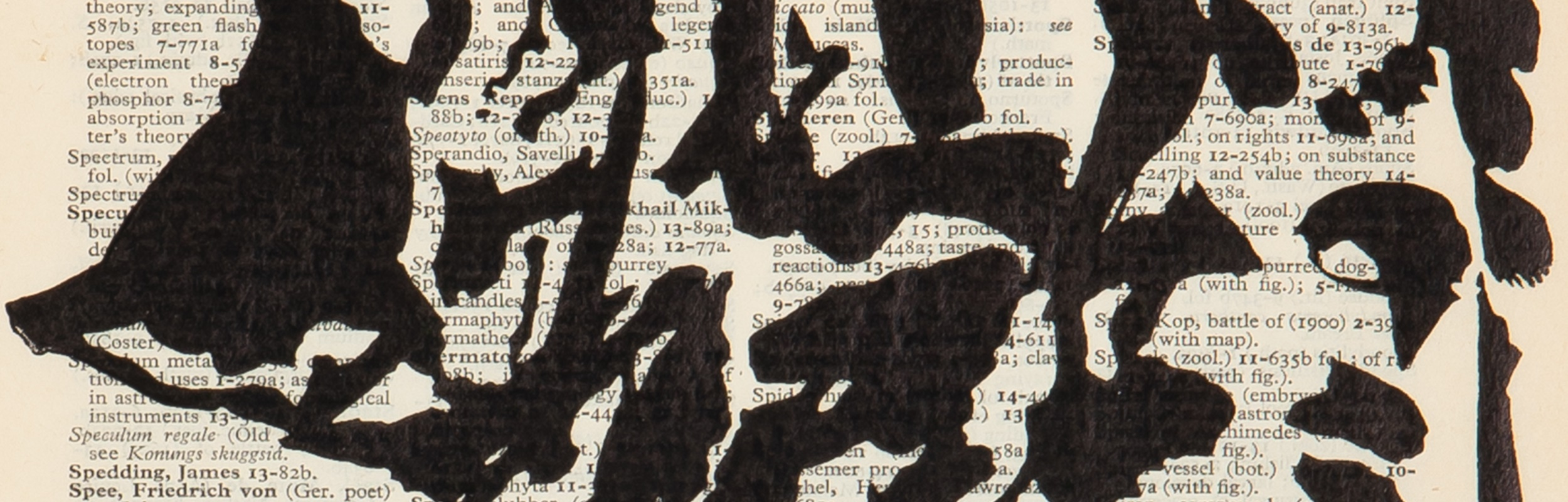##### Contemporary Art
starts: 17th Aug, 2023 11:00
Ends: 29th Aug, 2023 18:00

Name: Ms Carina Jansen
Email: carina@aspireart.net
Telephone: +27 78 968 2476

Name: Ms Mtha Poni
Email: mtha@aspireart.net
Telephone: +27 21 418 0765

Category

Estimated at R12,000 - R18,000

Sold for R3,986

Estimated at R3,000 - R4,000

Estimated at R18,000 - R24,000

Sold for R16,415

Estimated at R15,000 - R20,000

Sold for R15,242

Estimated at R6,000 - R8,000

Sold for R12,898

Estimated at R3,000 - R5,000

Sold for R3,518

Estimated at R3,000 - R4,000

Sold for R3,752

Estimated at R3,000 - R4,000

Estimated at R6,000 - R8,000

Sold for R11,725

Estimated at R12,000 - R16,000

Sold for R21,105

Estimated at R20,000 - R30,000

Estimated at R12,000 - R15,000

Sold for R58,625

Estimated at R50,000 - R70,000

Estimated at R40,000 - R60,000

Sold for R32,830

Estimated at R15,000 - R20,000

Estimated at R40,000 - R60,000

Sold for R70,350

Estimated at R60,000 - R80,000

Sold for R16,415

Estimated at R8,000 - R12,000

Sold for R9,380

Estimated at R8,000 - R12,000

Sold for R11,139

Estimated at R8,000 - R12,000

Sold for R9,380

Estimated at R8,000 - R12,000

Estimated at R35,000 - R50,000

Sold for R14,070

Estimated at R15,000 - R25,000

Sold for R1,876

Estimated at R2,000 - R3,000

Sold for R7,035

Estimated at R6,000 - R9,000

Estimated at R20,000 - R30,000

Sold for R23,450

Estimated at R20,000 - R30,000

Estimated at R30,000 - R40,000

Estimated at R45,000 - R55,000

Estimated at R30,000 - R40,000

Sold for R23,450

Estimated at R20,000 - R30,000

Sold for R35,175

Estimated at R30,000 - R40,000

Estimated at R6,000 - R8,000

Estimated at R50,000 - R80,000

Sold for R9,380

Estimated at R10,000 - R15,000

Estimated at R15,000 - R25,000

Estimated at R8,000 - R12,000

Sold for R55,750

Estimated at R60,000 - R80,000

Estimated at R10,000 - R15,000

Sold for R37,520

Estimated at R30,000 - R50,000

Estimated at R30,000 - R40,000

Sold for R10,552

Estimated at R18,000 - R24,000

Estimated at R15,000 - R20,000

Sold for R8,794

Estimated at R8,000 - R12,000

Estimated at R25,000 - R35,000

Estimated at R8,000 - R12,000

Estimated at R25,000 - R35,000

Estimated at R15,000 - R20,000